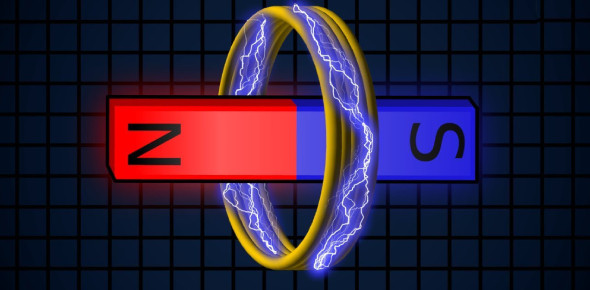# 4th Grade: Electricity And Magnetism Test! Trivia Quiz

13 Questions | Total Attempts: 989SettingsEver wondered how much is expected from you to know about electricity and magnetism as a fourth-grader? Electricity and magnetism are two related items produced by an electromagnetic influence. A shifting electric charge produces a magnetic field, which incites electric charge movement, which creates an electric current. You must know the answers to what lightning a discharge of is and the complete path through which energy flows. You must take this energizing quiz.

• 1.
Lightning is a discharge of
• A.

Magnetic field

• B.

Direct curent

• C.

Static electricity

• D.

Chemical energy

• 2.
A complete path through which electricity flows is a(n)
• A.

Circuit

• B.

Series

• C.

Cell

• D.

Fuse

• 3.
The plastic around an electrical wire that keeps you from getting a shock is an example of a(n)
• A.

Conductor

• B.

Fuse

• C.

Insulator

• D.

Circuit breaker

• 4.
A thin wire strip that melts easily to keep too much electric current from flowing through wires is a(n)
• A.

Insulator

• B.

• C.

Fuse

• D.

Circuit

• 5.
To make light, a flashlight needs a bulb, a switch, and a(n)
• A.

Parallel

• B.

Insulator

• C.

Cell

• D.

Current

• 6.
A crane in a junkyard can separate iron and steel from paper using a(n)
• A.

Generator

• B.

Volt

• C.

Electromagnet

• D.

Transformer

• 7.
A device that is used to change the voltage of electricity is a(n)
• A.

Generator

• B.

Volt

• C.

Electromagnet

• D.

Transformer

• 8.
A unit for measuring the force that makes negative charges flow is called a(n)
• A.

Generator

• B.

Volt

• C.

Electromagnet

• D.

Transformer

• 9.
A magnet’s pull is strongest at its
• A.

Volt

• B.

Poles

• C.

Field

• D.

Generator

• 10.
A bulb gets dimmer when a second bulb is added to the circuit.  The bulbs are wired in a(n)
• A.

Series circuit

• B.

Parallel circuit

• 11.
A material electricity flows through easily is a(n)
• A.

Insulator

• B.

Fuse

• C.

Conductor

• D.

Resistor

• 12.
Which is NOT an example of the uses of an electromagnet?
• A.

Separating scrap iron from other garbage

• B.

Ringing a door bell

• C.

Finding which direction is North

• D.

Picking metal splinters out of skin

• 13.
A balloon sticks to a wall after being rubbed on a sweater because of
• A.

Magnetism

• B.

Static electricity

• C.

Mechanical energy

• D.

Chemical energy

Related TopicsBack to top